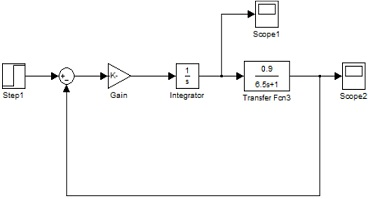# Explain the use of practical and analytical methods in creating and testing a measurement system

Industrial Systems - Systems Modelling

Learning Outcome 1: Apply practical and computer-based methods to design and test a measurement system

Learning Outcome 2: Apply practical and computer-based methods to design and test a measurement system

Learning Outcome 3: Explain the use of practical and analytical methods in creating and testing a measurement system

Learning Outcome 4: Interpret the characteristics and behaviour of an existing electronic measurement system by applying a variety of methods to find a solution to a given problem

Learning Outcome 5: Critically evaluate the performance of an ideal measurement system compared to a real circuit

Introduction

The speed and efficiency of many industrial processes is due, largely, to the control systems selected for the application and the engineer`s ability to apply the most appropriate technology for their operation.

This unit presents a structured approach to the development of advanced electronic solutions in a range of industrial situations. An essential requirement here is the engineer`s ability to utilise the most appropriate technology for each application, to ensure the most efficient monitoring and control of variables such as pressure, temperature, and speed.

Among the topics included in this unit are techniques and applications of electrical and electronic engineering, as they apply to various branches of industry, such as component handling, controlling the speed or torque of a motor or responding to change of circumstances in a process.

On successful completion of this unit students will be able to describe system elements and consider their overall characteristics. This provides opportunity for analytically assessing the accuracy and repeatability of electronic instruments.

Scenario

As a control engineer you are responsible for designing and implementing control systems that ensure that process or systems are optimised and behave in a desired way. To analyse and design stable systems you are required to employ simulation software and programming languages such as MATLAB or Simulink. Use your knowledge of computer-based tools to answer the following questions.

A (i) Explain the objectives of a control system and provide examples of systems where control is necessary to achieve these objectives.

Describe with the aid of sketches the following three types of control inputs that can be used in Simulink simulations and explain the advantages and disadvantages of each type.
Step
Impulse
Ramp

For the following characteristic equations, state if the system is stable, unstable, or marginally stable. Give the brief reason for your answer.

s3 - s2 + 2s + 5 = 0

s4 + 5s3 + 3s2 + 10s = 0

(s +3)(s+1)(s+5)(s+2)(s+0.3) = 0

Describe with the aid of relevant diagrams the following three characteristics of a first order transfer function.

Time constant
Settling time
Rising time

For the electric circuit shown in Figure 1 derive an ordinary differential equation expressing the output voltage V0(t) in terms of L, R, C and the applied voltage Vi(t). List the assumptions you have made.

Convert your answers from above into the generalised form for a 2nd order model, and use this to identify the forcing function, steady state gain, damping ratio and natural frequency. Briefly comment on how such generalised forms can be useful in the design and test of a measurement system.

A cruse control system of a toy car is designed to maintain a specific speed on the road by automatically adjusting the force imparted by the engine. Once the desired speed is reached the system should maintain that speed. Consider a control system with a step input and of first order differential equation.

τ.dx/dt + x = Ku

x is the speed (miles/hour), u is the engine force,
K is the steady state gain and τ is the time constant (seconds).

In this model, the engine force is represented as a percentage, where 100% implies full power, 50% half power and so on.

Solve this differential equation and prove that it will give the following Transfer function.

X = K/(τ+1)xU

(a) Construct and annotate a Simulink diagram of the above model. Plot the response of the model to a step-in engine power from zero to 50 percent power, with the step occurring after 10 seconds and assuming zero initial conditions. Submit the Simulink diagram and your graph.

To improve the control system an integrator and feedback have been included in the design as below.Determine the percentage overshoot of the new system.

Using trial and error determine the appropriate value of the gain that will give a good response. (That is one with minimum overshoot and no oscillation)

By Critically analysing the above control provide reasons why the introduction of an integral control will improve the performance of this system.

Critically evaluate the performance of this speed control system compared to a real designed car system.